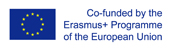This project has been funded with support from the European Commission.
This web site reflects the views only of the author, and the Commission cannot be held responsible for any use which may be made of the information contained therein.

Select language   >   IT EN RO PL LT

### Probability

Subject taught
- Math

Type of Product
- Web Site/Portal

Language Skills Developed
- Speaking
- Writing

Transferable/Scientific Skills Developed
Student will develop self-motivation, analytical skills, problem-solving skills.

Description
General aims:

Student will be able to determine the probability of an event using the results of an experiment. Student will be able to solve basic mathematical problems connected with probability which can be used in the real life. He will know the language and patterns associated with probability. Student will develop positive learning habits such as organisational and problem-solving skills.

Linguistic aims:
- to read English with ease
- to understanding content
- to learn from written texts
- to master tools for gathering information
- to express understanding and experiences
- to enable students to understand topic correctly
- to translate words connected with probability in English into the mother tongue.

Subject specific aims:
- to familiarise students with the ways in which we talk about uncertainty
- to engage students in activities which will give them contact with the ideas of probability
- student is able to determine the probability of an event using the results of an experiment
- student is able to use a scale from 0 to 1 to informally place everyday chance-related events
- represent and interpret probabilities as fractions or decimal.

Target group age:
Students from primary school 13-14 years old. What is more, this web site can be used by anyone who wants to do extend one’s knowledge about probability .

Level of competence in English (CEFR)
B1/B1+

Time required to use the resource with the students:
The material can be done in 45 minutes. If the teacher wants to introduce the types of events than she or he will need one more lesson.

How to use it
The teacher can ask the students to familiarize themselves with the website at home. In this part the students get to know activities which will give them contact with the ideas of probability.

Students should try to understand the text in English as well as the concept of probability and the content of the website.

During lessons the teacher can ask the student in English:
“What is the chances of rolling a "2" with a die ?”,
“A die is thrown once. What is the probability that the score is a number 6?”,
“If I say that the probability »of one of you flying to Moon tomorrow is 5 – is this possible?”

The students give the answers with the explanation. The teacher should point the good answers and show similar examples from the resource to visualize the most important issues and definitions for example “a probability line,” “experiment,” “outcome,” “sample space,” “sample point,” the formula how to calculate “probability of an event happening”. The teacher highlights some new words for students in English and students should find the translation of these words into the mother tongue and write down the new words in English in the notebook with the right translation. The teacher should also highlight what it means in mathematics when we say "fair dice," and ask students to write down the plural of die (dice).
Then, the students do the basic examples where they have to determine the probability of an event using the results of an experiment.
They will also can solve more problems, there are questions from one to ten which students can do in pairs. The students can discuss the problems in pairs and after that they can try to express their conclusions and possible questions. The teacher comments and corrects their statements. Then, the student can solve similar math problems. After doing and checking the exercises teacher can ask students what they learn and ask them to write down in their notebooks new words in English.

It would be also recommended to give students more examples of experiments connected with ones presented in the resource. The teacher may need another lesson to finish the explanation of all examples from this section. There are extra activities in the resource:
• An experiment with a die
• An experiment with dice
• Dropping a coin onto a grid
• Buffon's needle
• Random words
• Lotteries
(https://www.mathsisfun.com/data/index.html#probability)
• Complement
• Probability: Types of Events
• Independent Events
• Dependent Events: Conditional Probability
• Tree Diagrams
• Mutually Exclusive Events
(https://www.mathsisfun.com/data/probability-events-types.html)
which can be used during lesson.
Possible difficulties for the students
Students who have poor knowledge of English can have problems with understanding the content. Students who has problems doing basic operations with fractions, decimals and percentages can face difficulties in solving math problems connected with the probability which are introduced on the web site which is recommended.